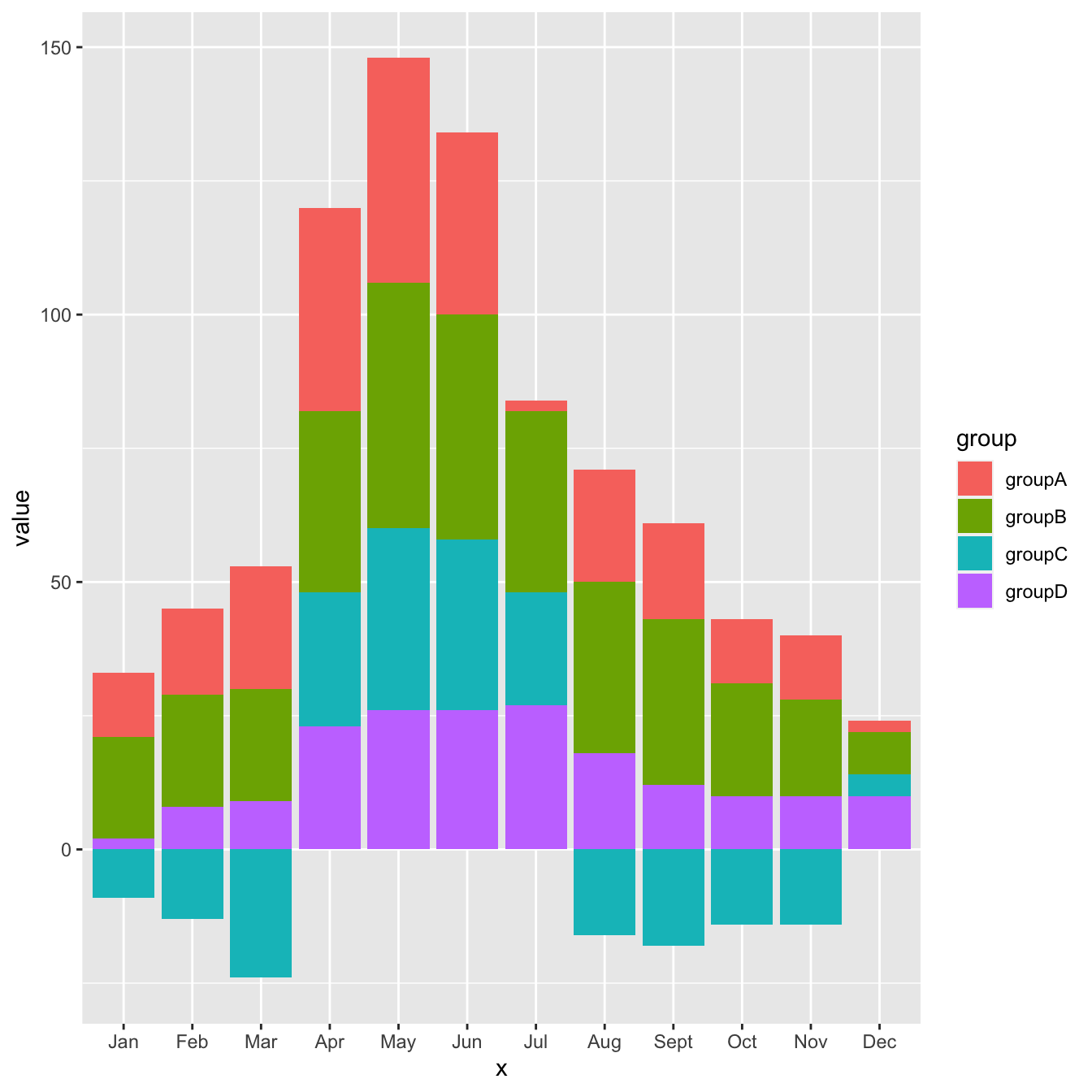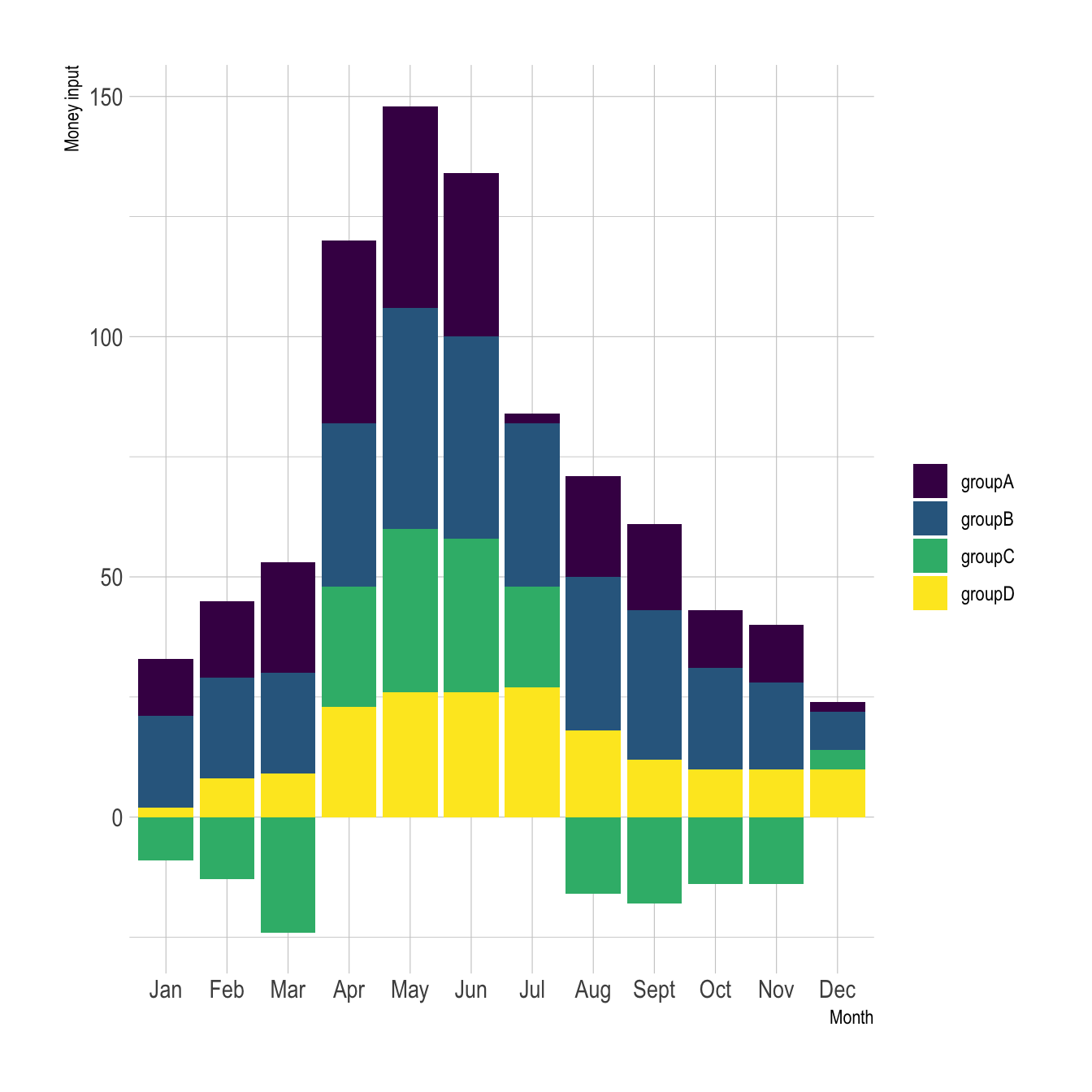# Stacked barplot with negative values with ggplot2

This post explains how to build a stacked barplot with R and ggplot2. It focus on a dataset that includes negative values

# Dataset

The dataset used in this post has 5 columns:

• the first column is called `x` and actually provides the month of the year, from January to December
• the columns 2 to 5 provide a numeric value for 4 employees of a company respectively. Let’s say it’s the amount of money they brought or spend each month.

The dataset is stored on github and can be loaded as follow:

``````# Load the dataset that is stored on the web

This is an overview of how it looks like:

``````library(knitr)
x groupA groupB groupC groupD
Jan 12 19 -9 2
Feb 16 21 -13 8
Mar 23 21 -24 9
Apr 38 34 25 23

To work with `ggplot2` and more generally with the `tidyverse`, it’s necessary to transform it to a “long” or “tidy” format. This is totally doable thanks to the `tidyr` package:

``````# Load the package
library(tidyr)
library(dplyr)

# transform the format
data_long <- gather(data, group, value, groupA:groupD) %>%
arrange(factor(x, levels = c("Jan", "Feb", "Mar", "Apr", "May", "Jun", "Jul", "Aug", "Sept", "Oct"))) %>%
mutate(x=factor(x, levels=unique(x)))

# And that's the result!
x group value
Jan groupA 12
Jan groupB 19
Jan groupC -9
Jan groupD 2

Note: sorting the data is annoying and is described more in depth here

# Stacked barchart

It’s now pretty straightforward to build the stacked barplot. Pretty much the same guideline that this specific post are applied. It’s important to notice that the negative values are automatically located below the 0 baseline, without any additional work required.`````` # library
library(ggplot2)

# plot
ggplot(data_long, aes(fill=group, y=value, x=x)) +
geom_bar(position="stack", stat="identity")``````

# Stacked barchart polish

As always, a few polish steps to make the chart looks better with proper axis titles, better theme and a classic `viridis` color palette.`````` # library
library(ggplot2)
library(hrbrthemes)
library(viridis)

# plot
ggplot(data_long, aes(fill=group, y=value, x=x)) +
geom_bar(position="stack", stat="identity") +
scale_fill_viridis(discrete=TRUE, name="") +
theme_ipsum() +
ylab("Money input") +
xlab("Month")``````

# Conclusion

By default, `ggplot2` uses a “diverging” approach when it comes to stacking negative values. Basically, it places all the negative values below the 0 axis, and all the positive ones above.

If stacking is a topic that interests you, this article goes more in depth.

Related chart types

## Contact

This document is a work by Yan Holtz. Any feedback is highly encouraged. You can fill an issue on Github, drop me a message on Twitter, or send an email pasting yan.holtz.data with gmail.com.